# Mathematics - Mathematical Analysis Vectors, Lines and Planes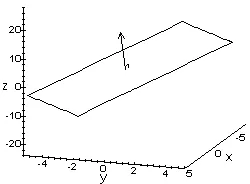Source

## Introduction

Hello it's a me again drifter1!

It's Easter Holiday time for me and so I got back home to chill for 2 weeks with my family and friends. In the first week I had some time for posting, but during the weekend we went to my Aunt and so I don't had time to post! But here I am again to  post for you Steemians!

Today's topic is Mathematical Analysis again and more specifically Vectors and equations for Lines and Planes! I already covered a lot about Vectors during my Physics "category" and so I suggest you to read the post about it here. We will make a quick recap and then continue with some more things that I didn't had to talk about in Physics! After that we will get into Lines and Planes...

So, without further do, let's dive straight into it!

## Quick recap

Vectors are arrows that point from one point to another and describe the direction of movement and also by how much we move in each of the x, y or even z coordinates in 1-, 2- or 3-dimensional space (meter).

From the Physics post (tweaked)...

Two vectors are equal when they have the same direction and meter.

The meter |A| of vector A is of course equal to: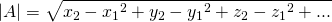via quicklatex

where A = (P1P2) with P1 = (x1, y1, z1, ...) and P2 = (x2, y2, z2, ...)

Vectors can be analyzed into components for each axis.

We can of course do some operatios on vectors that include:

• Addition -> A + B = C
• Substraction -> A - B = D
• Scalar product -> A*B = |A| |B| cosφ and so a real number
• Cross product -> AxB = ABsinφ and so a new vector [calculated using a determinant]

To have a common language we use unit vectors for each axis of a linear space, where an unit vector has meter 1.

To make a vector into a unit vector (meter 1) you simply divide it with it's meter!

## Vector tangents and normals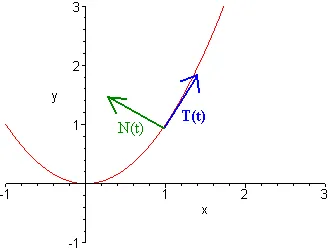Source

• A vector/line is tangent to an vector/line if they are parallel to each other.
• On the other hand it's a normal when they are vertically across.

To find a parallel (tangent) vector at a specific point of a line we simply find a unit vector with the same angle λ as the line. On the other hand for finding a normal vector we find a vector with angle -1/λ, but there is also a small trick if we already found the answer of the tangent.

Example

For the line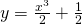(quicklatex) at x = y = 1 we have:
(quicklatex)

So, the vector we search for is:

v = 2i + 3j which has an angle of 3/2

The corresponding unit vector is:(quicklatex)

This is the tangent at (1, 1).

We could also use the negatve vector -u.

For the normal vector we simply find the negative inverse of u.

To do that we swap the places of the components and change the sign of one of them.

So, a normal vector is: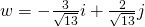(quicklatex)

Two more useful things are the following:

1. Two vectors are across if the scalar product of them is zero (u.v = 0).
2. Two vectors are parallel if the cross product of them is zero (uxv = 0)

## Vector projection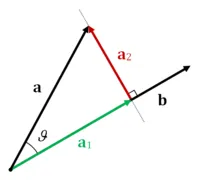Source

The vector projection of a vector u onto a line parallel to another non-zero vector v is the orthogonal projection of u straight into the line parallel to v.

From wikipedia

It can be noted as proj (u, v) which means projection of u onto v.

To calculate it we use:

proj (u, v) = |u|cosθ = u * v/|v|

where u*v is the scalar or dot product and θ the angle between u and v.

## Cartesian coordination space

The Cartesian space is defined using 3 axes (x, y and z).

Each point P is represented using values given to each of those coordinates that represent the position on each axis.

The center of the space is O(0, 0, 0).

In this space we can define planes like:

• xy where each point has z = 0
• xz where each point has y = 0
• yz where each point has x = 0

Using even more restrictions we can define more an more subspaces if this Cartesian space.

As already shown before vectors are represented using:

u = OP = xi + yj + zk

where (x, y, z) is the point P and i, j, k are the unit vector on each axis.

The meter is of course equal to the square root of the squares on of each coordinate.

Using the same concept we can calculate the distance between two points P1 = (x1, y1, z1) and P2 = (x2, y2, z2) in space, as the meter of the vector P1P2.

The equation of distance is:(quicklatex)

## Lines in space

A line in space can be represented by only two things:

• A point of the line (P)
• The slope/angle λ

A straight line is simply y = λx.

For more complex ones we use two representations:

1. Vector representation
2. Parametric representation

### Vector representation

A line L parallel to a vector v that surpasses the point P0 = (x0, y0, z0) is:

r(t) = r0 + i*v

where r is the position vector of the point P(x, y, z) of L and r0 is the position vector of the point P0.

### Parametric representation

By taking the components of the previous one we end up with the parametric representation.

For a line that contains the point P0 = (x0, y0, z0) and is parallel to the vector v = u1i + u2j + u3k we have:

x = x0 + t*u1

y = y0 + t*u2

z =  z0 + t*u3

where t is any real number.

So, to find a line we simply need to find a parallel vector to that line and need to know a point where the line passes by, cause the vector and line have the same slope/angle (tangent vector).

## Planes in space

Using the same concept we can also define a plane.

A plane that contains the point P0(x0, y0, z0) and is vertically across to a vector n = Ai +Bj + Ck (normal) is of the form:

n*(P0P) = 0

or

A(x - x0) + B(y - y0) + C(z - z0) = 0

or

Ax + By + Cz = D where D = Ax0 + By0 + Cz0

So, to find a plane we just have to find a normal vector to that plane and have a point given.

Because the Plane types are more advanced I leave them for a post on their own...

And this is actually it and I hope that you enjoyed it!

Bye!

H2
H3
H4
3 columns
2 columns
1 column